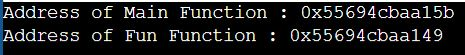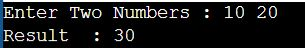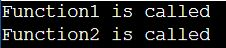# Function Pointer in C

## Function Pointer in C Language with Examples

In this article, I am going to discuss Function Pointer in C Language with Examples. Please read our previous articles, where we discussed Dangling Pointer in C Language with Examples.

##### Function Pointer in C Language

The pointer variable which holds the address of a function is called a function pointer. The basic advantage of a function pointer is that 1 function can be passed as a parameter to another function. Function pointer calls are faster than normal functions.

We already discussed that, in C Programming Language, we can create a pointer of any data type such as int, char, float, etc. It is also possible in C Programming Language that, we can create a pointer pointing to a function. In that case, the code of that function always resides in the memory. That means the function now has some address. We can get the address of memory by using the function pointer.

##### Example: Function Pointer in C Language

Let us understand Function Pointer in C Language with an example. In the below sample code, we are simply printing the address of the main and Fun functions.

```#include <stdio.h>
int Fun()
{
int x = 10;
}
int main ()
{
printf ("Address of Main Function : %p", main);
printf ("\nAddress of Fun Function : %p", Fun);
return 0;
}```
###### Output:From the above output, we can observe that the main() function as well as any other functions have some addresses. Therefore, we can also conclude that every function has some address.

##### Declaration of a Function Pointer in C Language:

In the previous example, we conclude that every function has some addresses. So, now, we will see how to create a pointer and how a pointer points to those function addresses.

Syntax of Function Pointer in C Language:
return_type (*ptr_name)(type1, type2…);

Example1: int (*IP) (int);
In this example, *IP is a pointer that points to a function that returns an integer value and accepts an integer value as an argument.

Example1: float (*FP) (float);
In this example, *FP is a pointer that points to a function that returns a float value and accepts a float value as an argument.

So, here, you can observe that the declaration of a function pointer is similar to the declaration of a function except that the pointer is preceded by a ‘*’. So, in the above two examples, FP and IP are declared as functions rather than pointers.

As of now, we have seen only how to declare a function pointer in C Language. Now. we will see how to assign the address of a function to a function pointer in C Programming Language.

##### How to assign the address of a function to a function pointer in C?

Let us see, how to assign the address of a function to a function pointer in C Language. The most important point that you need to remember is that the declaration of a function is necessary before assigning the address of that function to a function pointer. The following three statements describe the declaration of a function, declaration of a function pointer, and assigning the address of the function to a function pointer
Declaration of Function: int fun(int , int);
Declaration of a Function Pointer: int (*IP) (int , int);
Assigning the address of fun to the IP pointer: IP = fun;
In the above declarations, the function pointer IP contains the address of the fun function.

##### How to Call a function through a function pointer?

We already know how to call a function in C Language. Now, we will see how to call a function using a function pointer. Suppose we declare a function as follows:
int fun(int , int);

We can call the above function as follows:
int result = fun(10 , 20);

We can also call the above function using a function pointer as follows:
int result = (*IP)(10, 20); // Calling a function using function pointer.
OR
int result = IP(10,20); // Calling a function using function pointer by removing the indirection operator

Note: If we are using a function pointer to call a function in C Language, we can omit the indirection operator as we did in the second case. But, it is recommended to use the indirection operator as it makes it clear to the user that we are using a function pointer.

##### Program to Understand Function Pointer in C Language:
```#include <stdio.h>
int main()
{
int a, b;
int (*IP) (int, int);
printf ("Enter Two Numbers : ");
scanf ("%d %d", &a, &b);
int result = (*IP) (a, b);
printf ("Result  : %d", result);
return 0;
}

{
int c = a + b;
return c;
}```
###### Output:##### More Programs to Understand Function Pointer:
```#include<stdio.h>
void abc ()
{
printf ("Hello World\n ");
}

void main ()
{
void (*ptr) (void);
ptr = &abc;
ptr ();
}
```

Output: Hello World

##### Passing a function’s address as an argument to another function in C:

It is also possible in C Language that we can pass the address of a function as an argument to other functions in the same way we send other arguments to the function. Let us understand this with an example.

In the below example, we have created two functions, i.e., Function1 and Function2. The Function1 function contains the function pointer as an argument. In the main() method, the Function1 method is called in which we pass the address of Function2. When Function1 is called, the pointer ptr contains the address of the Function2 function. Inside Function1, we call the Function2 method by using the function pointer.

```#include <stdio.h>
void Function1(void (*ptr) ());
void Function2();
int main()
{
Function1(Function2);
return 0;
}

void Function1(void (*ptr) ())
{
printf ("Function1 is called");
(*ptr) ();
}

void Function2()
{
printf ("\nFunction2 is called");
}```
###### Output: